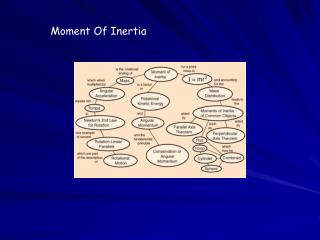DownloadDownload PresentationMoment Of Inertia

# Moment Of Inertia

Download Presentation## Moment Of Inertia

- - - - - - - - - - - - - - - - - - - - - - - - - - - E N D - - - - - - - - - - - - - - - - - - - - - - - - - - -
##### Presentation Transcript

1. Moment Of Inertia

2. Where does moment of inertia originate? • Angular acceleration is proportional to torque. • This corresponds to Newton’s law for translational motion’ • (where Force is replaced by torque and angular acceleration • takes the place of linear acceleration) • 3. In a linear case the acceleration is proportional to the Force • and inversely proportional to the mass.

3. Newton's first law of motion says "A body maintains the current state of motion unless acted upon by an external force." The measure of the inertia in the linear motion is the mass of the system and its angular counterpart is the so-called moment of inertia. The moment of inertia of a body is not only related to its mass but also the distribution of the mass throughout the body. So two bodies of the same mass may possess different moments of inertia.

4. It appears in the relationships for the dynamics of rotational motion. The moment of inertia must be specified with respect to a chosen axis of rotation. For a point mass the moment of inertia is just the mass times the square of perpendicular distance to the rotation axis, I = mr2. That point mass relationship becomes the basis for all other moments of inertia since any object can be built up from a collection of point masses.

5. Relationship of formulas F = ma and atan = ra so F = mra t = Fr so t = mr2a Here we have a direct relation between the angular acceleration and the applied torque. The quantity mr2 represents the rotational inertia of the particles of a certain mass rotating in a circle of radius “r” about a fixed point.

6. Common Moments of Inertia The moment of inertia of a system describes how the mass is distributed around the rotating object.

7. Which object will reach the bottom of the incline first? They have the same radius and are the same mass By calculating a value for “m” and ”R” you can see the hoop has a larger moment of inertia and therefore requires mrre energy to get it started.

8. Newton’s Second Law for rotation is seen below as it is compared to the linear second law. Also you can see how moment of inertia is used to compare linear and angular values for momentum, kinetic energy, and work

9. Rotational Kinetic Energy An object rotating around an axis is said to have rotational kinetic energy. The equation to the right represents the kinetic energy of a rigid rotating object.

10. An object that rotates while its center of mass undergoes translational motion will have both translational and rotational kinetic energies. Therefore the total kinetic energy of an object that rotates and moves in a linear direction is the sum of the rotational and translational kinetic energies. KE = ½ mv2 + ½ Iw2 If an object rolls down an incline the potential energy (mgy) = PE is converted to both rotational and translational kinetic energies But if an object slides down an incline all the potential energy is converted to kinetic energy

11. So using the kinetic energy equation which object will be moving the fastest at the bottom of the incline? Would a sliding object beat both these objects?

12. Work done by torque Work done on an object rotating about a fixed axis, such as a pulley can be written in angular quantities. W = Fr = FrDq since t = rF then W = tDq Power = W/Dt = tDq/Dt = rw

13. Angular momentum and its conservation In a like manner, the linear momentum, p=mv, has a rotational analog. It is called angular momentum, L. For an object rotating about a fixed axis, it is defined as; L = Iw Newton’s second law for rotation can also be written as; St = D L/ Dt

14. Angular momentum is an important concept in physics because, under certain conditions is a conserved quantity. We can see if the net torque on an object is zero, then the sum of the torques equals zero. That is, L does not change. This is the law of conservation of angular momentum for a rotating object – the total angular momentum of a rotating object remains constant if the net torque acting on it is zero.

15. http://hyperphysics.phy-astr.gsu.edu/hbase/inecon.html http://kwon3d.com/theory/moi/moi.html http://motivate.maths.org/conferences/conf14/c14_talk4.shtml